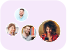Product Edition:5th Edition
Author: Stormy Attaway Ph.D. Boston University
Book Name: MATLAB: A Practical Introduction to Programming and Problem Solving
Subject Name: Maths

# MATLAB: A Practical Introduction to Programming and Problem Solving 5th Edition Solutions

0 out of 5.0
54 reviews45 Students
have requested for homework help from this book

MATLAB: A Practical Introduction to Programming and Problem Solving winner of TAA�s 2017 Textbook Excellence Award ("Texty") guides the reader through both programming and built-in functions to easily exploit MATLAB's extensive capabilities for tackling engineering and scientific problems. Assuming no knowledge of programming this book starts with programming concepts such as variables assignments and selection statements moves on to loops and then solves problems using both the programming concept and the power of MATLAB. The fifth edition has been updated to reflect the functionality of the current version of MATLAB (R2018a) including the addition of local functions in scripts the new string type coverage of recently introduced functions to import data from web sites and updates to the Live Editor and App Designer. Presents programming concepts and MATLAB built-in functions side by side giving students the ability to program efficiently and exploit the power of MATLAB to solve technical problemsOffers sections on common pitfalls and programming guidelines that direct students to best practice proceduresTests conceptual understanding of the material with Quick Questions! and Practice sections within each chapter NEW TO THE FIFTH EDITION Use of MATLAB Version R2018aA revised Text Manipulation chapter which includes manipulating character vectors as well as the new string typeIntroduction to alternate MATLAB platforms including MATLAB MobileLocal functions within scriptsThe new output format for most expression typesIntroduction to the RESTFUL web functions which import data from web sitesIncreased coverage of App DesignerIntroduction to recording audio from a built-in device such as a microphoneModified and new end-of-chapter exercisesMore coverage of data structures including categorical arrays and tablesIncreased coverage of built-in functions in MATLABRead more

5
27
4
11
3
11
2
11
1
0

0

## Students who viewed this book also checked out